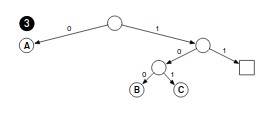# A nearly-trivial-on-zero-inputs 32-bytes-long collision-resistant hash function

The Libra whitepaper released yesterday also suggests a strategy for preventing the need to compute 256 hashes per Merkle proof in a sparse Merkle tree. Basically, subtrees consisting of exactly one leaf are replaced with a single node.This does arguably does increase the complexity of the sparse Merkle tree interface; their implementation is 460 lines of code.

In Compact Sparse Merkle Tree this approach is discussed as well

3 Likes

The proposed approach has two suboptimalities:

1. It still computes the hash every 16 steps.
2. The hash technically only uses 30 bytes, not 32.

For each node we compute a tuple (H, A), and at the root (assuming root always has both children) A is discarded. << below means cyclical shift.

1. If l \ne 0 \land r \ne 0 then (H, A) = (sha256(H(l) << A(l), H(r) << A(r)), 1)
2. If l = r = 0 then (H, A) = (0, 1)
3. If l = 0 then (H, A) = (H(r), 2A(r))
4. If r = 0 then (H, A) = (H(l), 2A(l) + 1)

It only produces shareable hashes (A = 1 and thus can be discarded) for nodes that have both children, which is generally the case for root I assume, and we usually don’t share hashes that are not the root of the tree?

A is effectively a bitmask of left and right turns taken going up on the chain of single children. Such a mask naturally cannot exceed 256 bits (since we take at most 255 steps as we go up to the root, and there’s leading 1), so shifting by A is always possible.

A particular hash that we feed into sha256 in (1) is the last computed sha256 shifted by such bitmask. For collision to occur here two sha256's need to be cyclical shifts of each other, which appears unlikely.

That has the issue that your hash is effectively 64 bytes, so it doesn’t fit neatly into the format of a 32-byte merkle tree. That’s roughly the direction I was going for before I figured this newer approach outI don’t think reducing hash security to 2**120 (or even 2**112 if we want to cut the hash overhead further from 16 to 8) is a particularly big deal. The main thing I dislike about this scheme is just the fact that it requires using a “different hash function” so it doesn’t cleanly with eg. other SSZ structures.

Lets look at matrix operations as the basis of a ‘hash function’ then.

You want to verify that H(x,0) != H(0,x), or … do you want that to be true? Where the hash function retains the associative and commutative properties of some underlying field.

Using matrix operations we can construct a function which returns the same result regardless of which side the input is on, if we say Ax is a matrix-vector-product, where you have some uniformly randomly chosen matrix A within a field.

Then you have x and y, which represent x=(a,0) and y=(0,a), you want Ax = Ay… This means that both of the inputs may be independent, but the sum of the two inputs equal the output.

Lets simplify this first, and provide a clearer example:

a(0) + b(x) = a(x) + b(0)

Which implies a and b are identical, where the sum evaluated at two points is also equal for either. Where a(0) = b(0) = 0 rite, and a(x) = b(x) = x?

So… lets take this back to matrix operations, where we want to directly translate the above statements into a problem which is ‘hard to solve’, and hopefully cryptographically secure…

Lets say x and y are our two inputs, where x is left and y is right. We need a matrix where the vector product relation exists such that 0 || x = y || 0 = x || 0 = 0 || y.

So, A\cdot({\vec{x}+\vec{0}}) = A\cdot({\vec{0}+\vec{x}})

This preserves the commutative relationship, albeit over a matrix and a vector, which is kinda what we need… Even if the matrix A is a Cauchy matrix, MDS matrix, or pretty much any other kind of matrix we retain the commutative and associativity laws when operating over a finite field.

Take, for example, the following Sage code:

from random import randint
q = 1244142437461793964053
n = m = 123 # for example
G = GF(q)
A = MatrixSpace(G, n, m).random_element()
RandomBinaryVector = lambda length: vector(G, [randint(0,1) for _ in range(length)])
x, y = RandomBinaryVector(n), RandomBinaryVector(n)
zero = vector(G,  * n)
assert A*(zero + x) == A*x
assert A*(y + zero) == A*y


However, the problem is… how do we make this not only cryptographically secure, but efficient…

At the moment it is neither…

With a hash function you would normally want to preserve the order of the inputs, so H(a,b) != H(b,a), but with a function which is cryptographically secure and preserves the commutative and/or associative relationships… you’re seeking a primitive operation which isn’t widely used… not only is it not widely used, but my personal analogy is that you might as well be juggling chainsaws when you’re dealing with polynomials and any hope of ‘security’ - especially when all commutative and associative relationships are preserved.

You can truncate a matrix down to a single field element, which gives you a transform of n\times m \to n \to 1 reduction, where the last step is the first element from the result, and the previous is a sum of some kind…

But… surely the whole ‘security argument’ about Poseidon and newer polynomial/matrix functions is that they disrupt the associative and commutative relationships, in the name of security.

1 Like

I definitely want H(a, b) != H(b, a), with it being computationally infeasible to find exceptions.